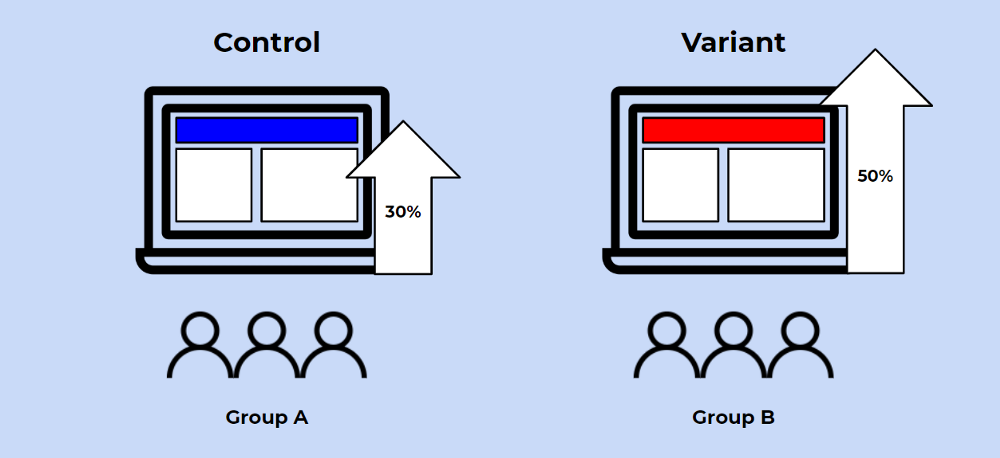1593337860

# A/B Testing with Chi-Squared Test to Maximize Conversions and CTRs

## Introduction

Arguably one of the most practical data science concepts in the workplace is A/B Testing. And yet, it is a concept that is quite misunderstood because there are a lot of intricacies to it.

To give an example, many experimentalists use the t-test to determine if there are significant differences between the two alternatives. But what if the distribution is not assumed to be Gaussian? What if the standard deviations of the two groups are different? What if the distribution is completed unknown?

In this article, I’m going to talk about a particular A/B Testing method that works well for comparing click-through rates and conversions.

## What is an A/B Test?

A/B testing in its simplest sense is an experiment on two variants to see which performs better based on a given metric. Typically, two consumer groups are exposed to two different versions of the same thing to see if there is a significant difference in metrics like sessions, click-through rate, and/or conversions.

Using the visual above as an example, we could randomly split our customer base into two groups, a control group and a variant (or treatment) group. Then, we can expose our variant group with a red website banner and see if we get a significant increase in conversions. It’s important to note that all other variables need to be held constant when performing an A/B test.

Getting more technical, A/B testing is a form of statistical and two-sample hypothesis testing. **Statistical hypothesis testing **is a method in which a sample dataset is compared against the population data. **Two-sample hypothesis testing **is a method in determining whether the differences between the two samples are statistically significant or not.

## Chi-Squared Test

What do click-through rates and conversions have in common? They have a Bernoulli Distribution, the discrete probability distribution that has a probability of being 1 and a probability of being 0. For click-through rates, a user will either click (1) or not click (0). Similarly, for conversions, a user will either convert (1) or not convert (0).

Because we’re performing an A/B Test on conversions which is a categorical variable that follows a Bernoulli distribution, we’ll be using the Chi-Squared Test.

The steps to conducting a chi-squared test are as follows:

1. Calculate the chi-squared test statistic
2. Calculate the p-value
3. Compare the p-value against the level of significance

This will make more sense when you follow along with the project walkthrough.

#programming #statistics #data-science #technology #testing #a/b testing